# Scalar Field & Its Gradient - Notes | Study Electromagnetic Fields Theory (EMFT) - Electrical Engineering (EE)

 1 Crore+ students have signed up on EduRev. Have you?

Concept of a Field :

By field, we basically mean something that is associated with a region of space. For instance, this room in which I am speaking can be considered to be a region in which a temperature field exists. Normally, we talk of the temperature of a room. However, this is in the sense of an average and does not provide detailed temperature profile inside the room. However, the temperature inside a room does vary from place to place. For instance, if you are in a kitchen, the temperature would be higher when you are close to stove and would be lower elsewhere. In principle, one can associate a temperature with every point inside the room. The field that we talked of here, viz. the temperature field is a scalar field because the field quantity “temperature” is a scalar.

The “field” is thus a region of space where with every point we can associate a scalar or a vector (it could be more generalized but for our purposes, these two will do). Coming to a vector field, as we know, a vector quantity has both magnitude and direction. Consider our room again. We can associate a gravitational field with it. Though we generally say that the acceleration due to gravity has a constant value inside the room, it is also meant in an average sense. In reality, its value and direction differs from place to place and a mass inside a room experiences a different force (both in magnitude and direction) depending on where in the room it is placed. If we talk of associating a force with every point in a certain region of space, we are talking about a vector field. In 2 dimensions, the force is a function of positions x and y and in three dimensions it is a function of x, y and z. Other than gravitational field, examples of vector fields are electric field and magnetic field.

Pictorially, the scalar field being defined by a number associated with a point in space ,is usually represented using a fixed spatial structure called a grid. They are also represented by connecting all points having the same value of the scalar field by a contour (e.g. isothermals). Since a vector field has a magnitude and direction, it is a little more complicated to represent it graphically. Let us consider a two dimensional vector field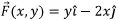as an example. We can use a graph paper with conventional x and y axes. How does one represent the vector field? We take some unit to represent a unit length of the vector field. In the figure below we have taken one fifth the unit spacing along x or y axes.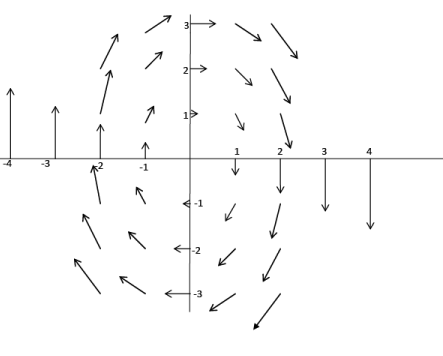Figure 1: Graphical representation of a vector field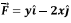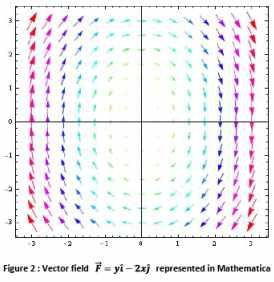In electrostatics we deal with force field due to charges. In Fig. 3 we show the force on a unit positive charge due to two equal and opposite charges . The vector field has been plotted at close enough points so that the field lines appear continuous. To find the force on a positive charge at a point, we need to draw a tangent to the field lines at that point. These are known as “lines of force” in electrostatics”. The arrow on the lines show the direction in which the charge moves.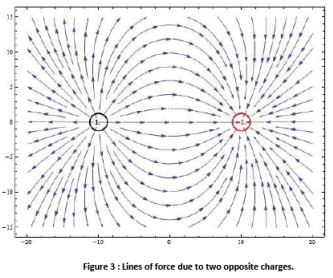Figure 4 shows the force field due to two similar charges, a positive charge is repelled by both the charges.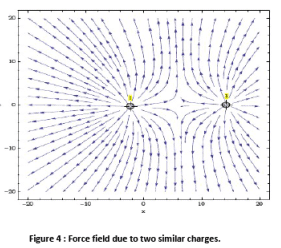Directional Derivatives :

Let me first remind you of the definition of ordinary derivative of a function f(x) of a single variable x. We define it by the relationship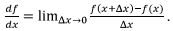This means that the value of the function f at the point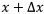is its value at the point x plus the derivative of the function time the increment in the value of x. If we are given a function in one dimension, the derivative at a point is the slope of the function at that point. If the slope is positive the value of the function increases from its value at a neighbouring point, it decreases if the slope is negative.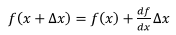What happens in higher dimensions? We are familiar with the concept of “partial derivative”. Suppose we have a function of x and y. The partial derivative with respect to x means that when the differentiation is done with respect to the variable x, we treat the variable y as a constant. Similarly, in taking partial derivative with respect to y, the value of x is kept fixed.

What if both x and y are to be allowed to vary simultaneously? The problem is that there are many ways the two variables can change simultaneously. Same is true for a function of three or more variables. The concept of derivative is thus to be generalized.

Suppose φ is a scalar function of the variables x, y and z. Starting from a point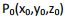if we move along an arbitrary direction by a length Δs, the value of the function at the destination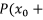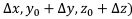will be given by its value at the initial point plus the derivative of the function computed along the direction in which we moved times the length Δs. Such a derivative is called the directional derivative. Since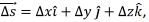we could go from the point P0 to the point P by going by a distance Δx along the x direction, keeping y and z constant, then going by an amount along the y direction and finally by Δz along the z direction and arrive at the point P.

This is graphically shown in two dimensions :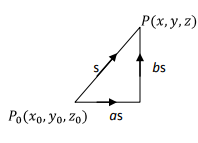Using the definition of partial derivatives, we have,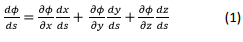where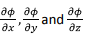respectively represent the partial derivatives of  φ  with respect to x, y and z respectively. Equation (1) gives the directional derivative of the scalar function φ along the direction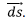Example : We will illustrate the concept of directional derivative by calculating the directional derivative of the scalar function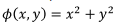along three different directions : along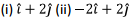and (ii)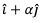at the point (1,2).

(i) The figure below shows the functionplotted along the z axis. It is a cup like structure.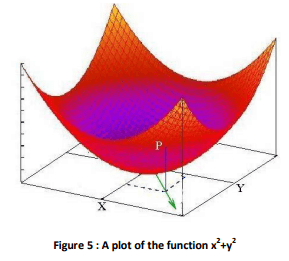Since the function is in two dimensions, we have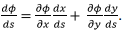The partial derivatives are given by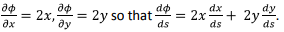In order to calculate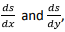we observe that along the given direction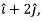the coordinates x and y are related by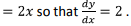We have ds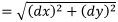which gives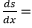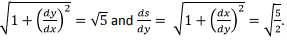Plugging these into the expression for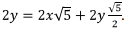At the point (1,2), the directional derivative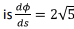(ii) The calculation is very similar to (i). The answer is zero.
(iii) Following the method outlined in (i) above, the directional derivative at the point (1,2) can be shown to be given by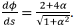The directional derivative has a maximum when α = 2. Thus the directional derivative at (1,2) has a maximum in the direction of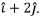It may be noted that this is the radial direction at that point.

Suppose the direction cosines of the direction that we move is (a,b,c), the unit vector in this direction represented by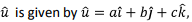with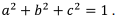We have,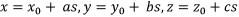, which gives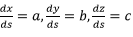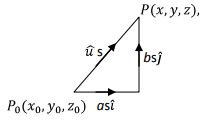Which results in the directional derivative along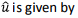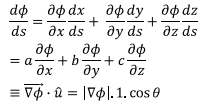Where the “gradient operator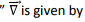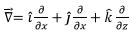where θ is the angle between the gradient and he direction in which the directional derivative is taken. Thus

(1) the magnitude of the gradient at a point is the maximum possible magnitude of the directional derivative at that point, and

(2) the direction of the gradient is that direction in which the directional derivative takes maximum value.

What does this physically mean? Suppose you are on a hill , not quite at the summit. If you want to come down to the base, there are many directions that you can take. Of all such possible directions, the fastest will be one which is steepest, i.e. with maximum slope.Since the rate of change in the value of the function is maximum along the gradient, it follows that such a direction is perpendicular to a surface on which the function is constant. Such a surface is called a “Level Surface”. Returning to the function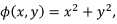level surface (rather a level curve in  this case) is the intersection of the plane z= constant with the surface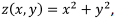which are family of circles. In Physics, the corresponding surface would be an equipotential surface and the direction of the gradient would correspond to the direction of the electric field.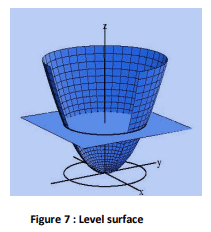In the present case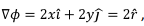which, as expected, is in the radial direction which is normal to the level curve, which is a circle

Gradient of a Scalar Field is a Vector Field and its direction is normal to the level surface.

Formal Proof : Consider a level curve which is parameterized by a variable t, which varies from point to point on the curve. Example of such a parameter for the circle is angle θ, so that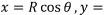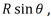where R is the radius (which is fixed) and q is the polar angle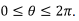The position vector of a point on the curve is given by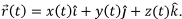Let the level curve be given by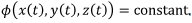The tangent to the curve is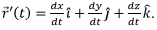Obviously, on the level curve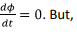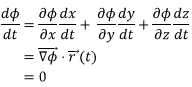Which shows that the gradient is normal to the level curve.

The document Scalar Field & Its Gradient - Notes | Study Electromagnetic Fields Theory (EMFT) - Electrical Engineering (EE) is a part of the Electrical Engineering (EE) Course Electromagnetic Fields Theory (EMFT).
All you need of Electrical Engineering (EE) at this link: Electrical Engineering (EE)

## Electromagnetic Fields Theory (EMFT)

11 videos|46 docs|62 tests
 Use Code STAYHOME200 and get INR 200 additional OFF

## Electromagnetic Fields Theory (EMFT)

11 videos|46 docs|62 tests

Track your progress, build streaks, highlight & save important lessons and more!

,

,

,

,

,

,

,

,

,

,

,

,

,

,

,

,

,

,

,

,

,

;